Test: SSBSC (Single Sideband Suppress Carrier) Transmission

# Test: SSBSC (Single Sideband Suppress Carrier) Transmission - Electronics and Communication Engineering (ECE)

Test Description

## 10 Questions MCQ Test GATE ECE (Electronics) 2024 Mock Test Series - Test: SSBSC (Single Sideband Suppress Carrier) Transmission

Test: SSBSC (Single Sideband Suppress Carrier) Transmission for Electronics and Communication Engineering (ECE) 2023 is part of GATE ECE (Electronics) 2024 Mock Test Series preparation. The Test: SSBSC (Single Sideband Suppress Carrier) Transmission questions and answers have been prepared according to the Electronics and Communication Engineering (ECE) exam syllabus.The Test: SSBSC (Single Sideband Suppress Carrier) Transmission MCQs are made for Electronics and Communication Engineering (ECE) 2023 Exam. Find important definitions, questions, notes, meanings, examples, exercises, MCQs and online tests for Test: SSBSC (Single Sideband Suppress Carrier) Transmission below.
Solutions of Test: SSBSC (Single Sideband Suppress Carrier) Transmission questions in English are available as part of our GATE ECE (Electronics) 2024 Mock Test Series for Electronics and Communication Engineering (ECE) & Test: SSBSC (Single Sideband Suppress Carrier) Transmission solutions in Hindi for GATE ECE (Electronics) 2024 Mock Test Series course. Download more important topics, notes, lectures and mock test series for Electronics and Communication Engineering (ECE) Exam by signing up for free. Attempt Test: SSBSC (Single Sideband Suppress Carrier) Transmission | 10 questions in 30 minutes | Mock test for Electronics and Communication Engineering (ECE) preparation | Free important questions MCQ to study GATE ECE (Electronics) 2024 Mock Test Series for Electronics and Communication Engineering (ECE) Exam | Download free PDF with solutions
 1 Crore+ students have signed up on EduRev. Have you?
Test: SSBSC (Single Sideband Suppress Carrier) Transmission - Question 1

### Which of the following modulation scheme is most bandwidth efficient?

Detailed Solution for Test: SSBSC (Single Sideband Suppress Carrier) Transmission - Question 1

The bandwidth of different modulation schemes are as shown:
AM → 2fm
SSB-SC → fm
For angle modulated schemes (FM and PM)
= 2(β + 1) fm
fm = message signal bandwidth
So, for SSB-SC, the bandwidth requirement is minimal.

Test: SSBSC (Single Sideband Suppress Carrier) Transmission - Question 2

### Direction: Two statements are given. Based on the given information, choose the correct answer. Statement-I: For the same message signal, the pre-detection SNR is double in the case of conventional practical AM modulation, than that in the case of SSB. Statement-II: The AM signal bandwidth is double as compared to SSB signal bandwidth.

Detailed Solution for Test: SSBSC (Single Sideband Suppress Carrier) Transmission - Question 2

Let, the channel is practical having finite attenuation of 'A' dB, and the single-sided noise power spectral density is given as 'N' W/Hz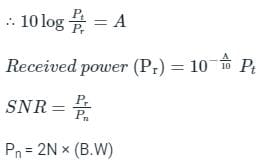Let the message signal spectrum is given as: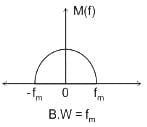In the case of AM:-

B.W = 2fm kHz (twice of message signal bandwidth)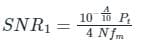In the case of SSB:-
B.W = fm kHz (same as message signal bandwidth)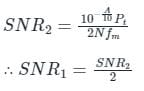∴ SNR in the case of S.S.B is double that in the case of AM.

Statement-I is incorrect and Statement-II is correct.

Test: SSBSC (Single Sideband Suppress Carrier) Transmission - Question 3

### The signal m(t) = cos(ωmt) is SSB (single side-band) modulated with a carrier cos(ωct) to get s(t). The signal obtained by passing s(t) through an ideal envelope detector is

Detailed Solution for Test: SSBSC (Single Sideband Suppress Carrier) Transmission - Question 3

1) General equation of SSB modulated wave: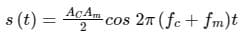2) Output of envelope detector to signal
Acos ωct → A
Application:
Equation of SSB modulated signal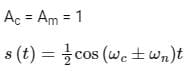the output of envelope detector = ½
The output of the envelope detector is a constant voltage signal
Hence option (d) which represents a constant voltage is correct.

Test: SSBSC (Single Sideband Suppress Carrier) Transmission - Question 4

Which of the following analog modulation scheme requires the minimum transmitted power and minimum channel bandwidth?

Detailed Solution for Test: SSBSC (Single Sideband Suppress Carrier) Transmission - Question 4

The frequency spectrum of all the given modulation schemes is as shown: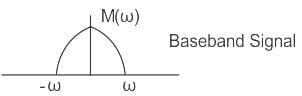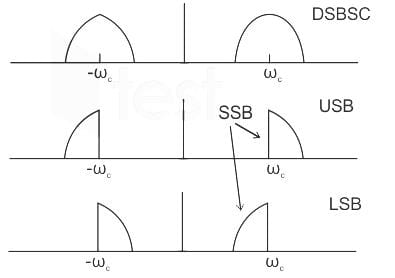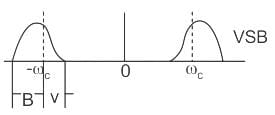Total power = Carrier power + Sideband power
In SSB, we have one sideband only hence the power is less than DSB and VSB.
In VSB, we have one sideband and some part of the other sideband and hence power is more than SSB and less than DSB.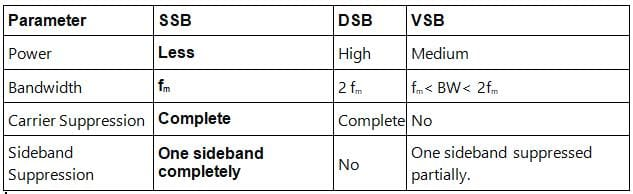Test: SSBSC (Single Sideband Suppress Carrier) Transmission - Question 5

What should be the bandwidth figure of signal to noise ratio for SSB?

Detailed Solution for Test: SSBSC (Single Sideband Suppress Carrier) Transmission - Question 5

Concept:
SSB (Single Side Band) modulation is a technique that allows only one sideband to pass saving bandwidth and power both.
Practically it is very difficult to pass only a single sideband.
Ex: If we consider a single-tone modulating signal as x(t) = cos ωmt, the SSB modulated wave with only the upper sideband will be represented as:
cos ((ωc + ωm)t) = cos ωc.cos ωmt – sin ωc.sin ωmt and with lower sideband only will be represented as: cos (ωc - ωm)t = cos ωct .cos ωmt + sin ωct. sin ωmt
where sin ωmt is the Hilbert transform of the original message signal x(t) = cos ωmt
Generalizing this for any signal m(t), we can say that the SSB modulated signal with only upper sideband will be: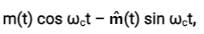Similarly, we can say that the SSB modulated signal with only lower sideband will be: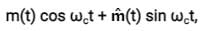where m̂(t) is the Hilbert transform of m(t).
The generalized AM expression is represented as:
s(t) = Ac [1 + μa mn (t)] cos ωct
The RF bandwidth of an SSB-AM signal is also 3 kHz.

Test: SSBSC (Single Sideband Suppress Carrier) Transmission - Question 6

What is the percentage of power saved when an 80% modulated wave is transmitted over SSB-SC which was initially transmitted over AM?

Detailed Solution for Test: SSBSC (Single Sideband Suppress Carrier) Transmission - Question 6

Concept:
Power of a transmitted AM wave is given as: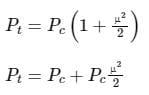Power in the carrier = Pc
Power in both the sidebands is given by: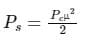Since the power is distributed equally to the left and to the right side of the sideband, the power in one of the sidebands is given by: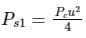Analysis:
In SSB-SC, the carrier and one of the sidebands are suppressed, and when the carrier and 1 of the sideband are suppressed, the amount of power saving will be: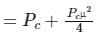The percentage of power saved will be calculated as: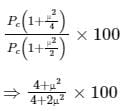Given μ = 0.80, the amount of power saving will be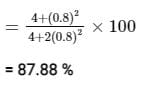Test: SSBSC (Single Sideband Suppress Carrier) Transmission - Question 7

The modulation technique that takes the lowest bandwidth among the given

Detailed Solution for Test: SSBSC (Single Sideband Suppress Carrier) Transmission - Question 7

The modulation technique that takes the lowest bandwidth is SSB-SC

Single Side Band (SSB SC):

• SSB SC is a form of amplitude modulation where only a single sideband is transmitted and 1 sideband and carrier are suppressed.
• The advantage of SSB is bandwidth and power-saving over both conventional AM and DSB SC, i.e. DSB SC has a more power consumption than SSB SC.
• The Bandwidth requirement is only fm, ∴ It needs half the bandwidth than the DSB-SC transmission.

Comparison: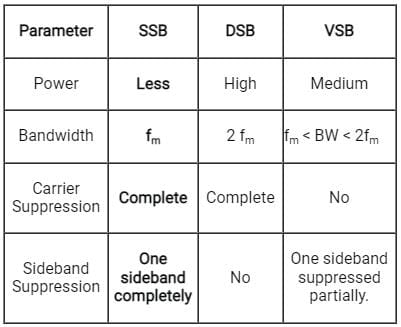Test: SSBSC (Single Sideband Suppress Carrier) Transmission - Question 8

Which of the following is NOT one of the types of amplitude modulation?

Detailed Solution for Test: SSBSC (Single Sideband Suppress Carrier) Transmission - Question 8

Vestigial Sideband:
VSB (vestigial sideband) transmission transmits one sideband fully and the other sideband partially thus, reducing the bandwidth requirement.
Vestigial Sideband Modulation (VSB) is used for video modulation in TV transmission.
The spectrum of a vestigial sideband is as shown: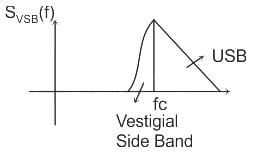Single Side Band (SSB SC)

• SSB SC is a form of amplitude modulation where only a single sideband is transmitted and 1 sideband and carrier are suppressed.
• In advantage of SSB is bandwidth saving and power saving over both conventional AM and DSB SC.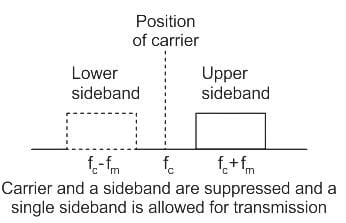Double Side Band (DSB SC):

• DSB -SC is a form of Amplitude modulation where only the sidebands are transmitted and carrier is suppressed.
• The advantage is 66% power saving compared to conventional AM.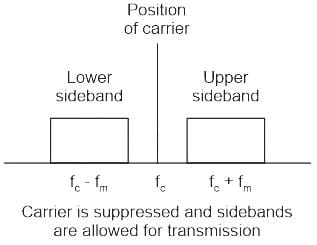Test: SSBSC (Single Sideband Suppress Carrier) Transmission - Question 9

Which of the following circuits could NOT be used as demodulator of SSB?

Detailed Solution for Test: SSBSC (Single Sideband Suppress Carrier) Transmission - Question 9

Phase Discrimination is used for the demodulation of Frequency Modulate signals.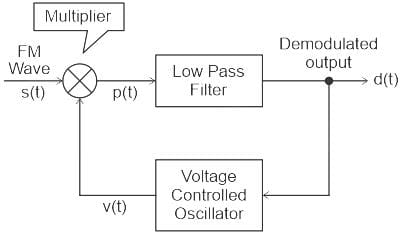Test: SSBSC (Single Sideband Suppress Carrier) Transmission - Question 10

How much percentage power will be saved when the carrier and one of the sidebands are suppressed in an AM wave modulated to a depth of 50% ?

Detailed Solution for Test: SSBSC (Single Sideband Suppress Carrier) Transmission - Question 10

Concept:
Total power in AM (PT)= PC( 1 + μ2/2 ) = PC + PCμ2/2
where;
PC → Carrier power
μ → modulation index
Power of single sideband = ( PCμ2/2)/2 =  PCμ2/4

Calculation:
Given
μ = 50% = 0.5
After suppressing carrier and a single sideband :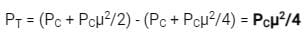Percentage of power will be saved :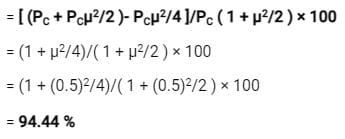## GATE ECE (Electronics) 2024 Mock Test Series

22 docs|263 tests
Information about Test: SSBSC (Single Sideband Suppress Carrier) Transmission Page
In this test you can find the Exam questions for Test: SSBSC (Single Sideband Suppress Carrier) Transmission solved & explained in the simplest way possible. Besides giving Questions and answers for Test: SSBSC (Single Sideband Suppress Carrier) Transmission, EduRev gives you an ample number of Online tests for practice

## GATE ECE (Electronics) 2024 Mock Test Series

22 docs|263 tests